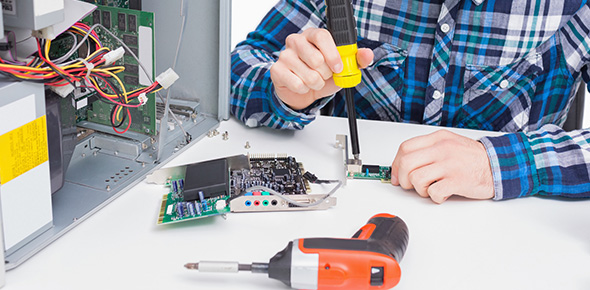# Quiz | Engineer's Day

52 Questions | Attempts: 125
ShareSettings• 1.
What does the IT abbreviation “D.O.S.” stand for?
• A.

Dry Ordering Service

• B.

Dual Operating System

• C.

Disk Operating System

• D.

Disk Ordering System

• 2.
What would you use VOIP to do on a computer?
• A.

Change Operating System

• B.

• C.

Make phone calls over the internet

• D.

Build a website

• 3.
A database format, what do the letters “S.Q.L.” stands for?
• A.

• B.

Structured Query Language

• C.

Structured Question Language

• D.

Shrewd Query Language

• 4.
Which of these is a method of photographing bones?
• A.

Alpha Rays

• B.

Gamma Rays

• C.

X-rays

• D.

Q-rays

• 5.
What is the smallest unit of a digital picture?
• A.

Pixel

• B.

Picpart

• C.

Decainch

• D.

Millimetre

• 6.
In navigation, what does the abbreviation “G.P.S.” stand for?
• A.

Greater Place Selector

• B.

Global Positioning System

• C.

• D.

Global People System

• 7.
Solar cells use _____ to turn light energy into electrical energy.
• A.

Electromagnetics

• B.

Semiconductors

• C.

Superelements

• D.

Superconductors

• 8.
Complete the missing words:The integration of large numbers of tiny __________ into a small chip was an enormous improvement over the manual assembly of circuits using electronic components.
• A.

Vacuum tubes

• B.

Transistors

• C.

Circuits

• D.

Devices

• 9.
The image below is an electrical symbol for ________ ?
• 10.
A solar cell can only absorb 15 to 25 percent of the sun’s energy because…?
• A.

Not all wavelength of light create the right reaction in the cell.

• B.

There’s too much resistance inside the semiconductor.

• C.

Both A and B

• 11.
What scientist is well known for his theory of relativity?
• A.

Planck

• B.

Einstein

• C.

Newton

• D.

Schrodinger

• 12.
The most commonly used semiconductor material is
• A.

Silicon

• B.

Germanium

• C.

Mixture of Silicon and Germanium

• D.

None of the above

• 13.
An OR gate has 4 inputs. One input is high and the other three are low. The output is
• A.

Low

• B.

High

• C.

Alternatively high and low

• D.

Alternatively high and low

• 14.
Both OR and AND gates can only have two inputs.
• A.

True

• B.

False

• 15.
Internally, a computer’s binary data are almost always transmitted on parallel channels, commonly referred as the:
• A.

Parallel bus

• B.

Data bus

• C.

Serial bus

• D.

Memory bus

• 16.
The check sum method of testing a ROM :
• A.

Indicates if the data in more than one memory location is incorrect

• B.

Provides a means for locating and correcting data errors in specific memory location.

• C.

Allows data errors to be pinpointed to a specific memory location.

• D.

Simply indicates that the content of the ROM are incorrect.

• 17.
• A.

• B.

• C.

• D.

• 18.
E = mc2 is a famous equation by Einstein where c equivalent to?
• A.

299,792,458 m/s

• B.

2.71828182846

• C.

1.61619997X10-35 meters

• D.

3.14159265359 units

• 19.
How many terminals does a Bi-polar Junction Transistor have?
• A.

3

• B.

4

• C.

2

• D.

1

• 20.
If four 9V batteries are connected series-aiding, the total is 36V.
• A.

True

• B.

False

• 21.
The unit of voltage is the ________ and the symbol for voltage is _____.
• A.

Joule, V

• B.

Coulomb, L

• C.

Volt, V

• D.

Volt, Q

• 22.
Javascript is a ________.
• A.

Client side scripting

• B.

Server side scripting

• C.

Markup language

• D.

• 23.
#FFFFFF form what color?
• A.

White

• B.

Black

• C.

Red

• D.

Green

• 24.
The internal impedence of an ideal current source is
• A.

Zero

• B.

Low

• C.

High

• D.

Infinity

• 25.
The type of transmission used for sound in TV transmission is
• A.

AM

• B.

FM

• C.

PCM

• D.

PWM

## Related TopicsBack to top
×

Wait!
Here's an interesting quiz for you.